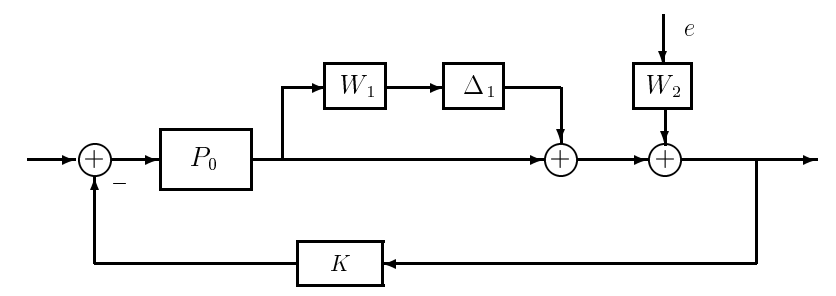# 21.2: Robust Disturbance Rejection

We will focus our discussion on one prototype problem, namely, the robust disturbance rejection problem shown in Figure 21.1. This motivates the following problem:

Robust Disturbance Rejection Projection (RP)

Find conditions on the nominal closed-loop system $$(P_{o}, K)$$ such that

1. $$K$$ robustly stabilizes all $$P \in \Omega$$, where $$\Omega=\left\{P \mid P=\left(I+\Delta_{1} W_{1}\right) P_{o}, \quad\|\Delta\|_{\infty}<1\right\}$$

2. $$\left\|(I+P K)^{-1} W_{2}\right\|_{\infty} \leq 1$$ for all $$P \in \Omega$$.

From Lecture 20, a performance objective in terms of the $$\mathcal{H}_{\infty}$$-norm of some closed loop map between some exogenous input $$w$$, to a regulated variable $$z$$, is mathematically equivalent to a robust stabilization problem with a perturbation block mapping the regulated output $$z$$ to the exogenous input $$w$$. Obviously, the new perturbed system is stable if and only if $$|| T_{z w} \|_{\infty} \leq 1$$, which is the performance objective. Notice that if the performance objective consists of several closed loop maps, then several perturbation blocks can be introduced in exactly the same fashion.Figure 21.1: Uncertain Plant with Disturbance

Proceeding for RP, we can "wrap" a frequency-weighted perturbation from the output to the input of interest, which results in the model of Figure 21.2. Next, we can re-arrange the system into the M-$$\Delta$$ feedback form (a nominal stable $$M$$ in feedback with the perturbation $$\Delta$$) as in Figure 21.3. In this case, however, there are multiple inputs and outputs to consider. We use the following procedure to generate $$M$$ and $$\Delta$$:Figure 21.2: Robust Performance Model

1. Define $$w_{i}, z_{i}$$ to be the output and input, respectively, of the perturbation $$\Delta_{i}$$.

2. For a total of $$m$$ perturbations, compute the matrix transfer function M as the map from

$w=\left[\begin{array}{c} w_{1} \\ \vdots \\ w_{m} \end{array}\right] \quad \text { to } \quad z=\left[\begin{array}{c} z_{1} \\ \vdots \\ z_{m} \end{array}\right]\label{21.2}$

In other words, all the $$\Delta$$ blocks are removed, and the transfer functions "seen" by the blocks from each input $$w_{j}$$ to each output $$z_{i}$$ are calculated and used as the $$(i, j)th$$ element of $$M$$.

3. The perturbation matrix $$\Delta$$ will have the structure

$\Delta=\left[\begin{array}{lll} \Delta_{1} & & \\ & \ddots & \\ & & \Delta_{m} \end{array}\right], \quad\left\|\Delta_{i}\right\|_{\infty}<1\label{21.2}$

For a SISO system, each $$\Delta_{i}(j \omega)$$ is a scalar, so that $$\Delta$$ becomes a diagonal matrix with complex entries. In the MIMO case, $$\Delta$$ is block-diagonal.

Example $$\PageIndex{21.1}$$ (Robust Disturbance Rejection)

Applying the robust performance procedure to Figure 21.2 yields:

$M=\left[\begin{array}{cc} -W_{1}\left(I+P_{0} K\right)^{-1} P_{0} K & -W_{1}\left(I+P_{0} K\right)^{-1} P_{0} K \\ W_{2}\left(I+P_{0} K\right)^{-1} & W_{2}\left(I+P_{0} K\right)^{-1} \end{array}\right]\label{21.3}$

The transfer functions on the diagonal are identical to those in the single-block robust stability and disturbance-rejection problems, respectively, while the off-diagonal terms account for the interaction between the two constraints. Having found the appropriate $$M$$ and $$\Delta$$, we have thereby reduced the robust performance problem to a stability problem for the system of Figure 21.3.Figure 21.3: M- $$\Delta$$ Feedback Form

A sufficient condition for robust stability is given by the small gain theorem, namely,

\begin{aligned} &\sigma_{\max }[M(j w)] \sigma_{\max }[\Delta(j w)] \leq \gamma<1\\ &\text { for all } w \text { . } \end{aligned}\nonumber

Since $$\Delta$$ is norm bounded by one, this condition translates to $$\|M\|_{\infty} \leq \gamma$$. This condition, however, is far from necessary since $$\Delta$$ has a block diagonal structure.